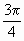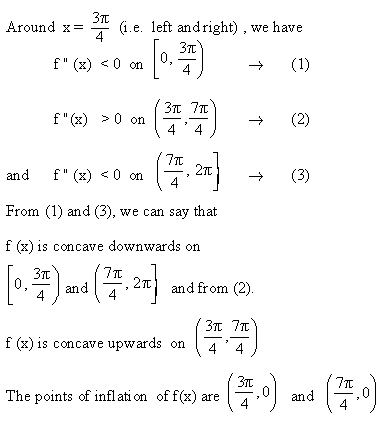Home MonkeyNotes Printable Notes Digital Library Study Guides Study Smart Parents Tips College Planning Test Prep Fun Zone Help / FAQ How to Cite New Title Request

Example 24

Determine the concavity of f (x) = cos x + sin x on [0, 2p] and identify any points of inflection.

Solution : f (x) = cos x + sin x and x Î [0, 2p]

Also, f  (x) = cos x - sin x and

f " (x) = - sin x - cos x

Now f " (x) = 0

Þ - sin x = cos x

\ tan x = -1

\ x =,Î [0, 2p]Your browser does not support the IFRAME tag.Index

5.1 Tangent And Normal Lines
5.2 Angle Between Two Curves
5.3 Interpretation Of The Sign Of The Derivative
5.4 Locality Increasing Or Decreasing Functions 5.5 Critical Points
5.6 Turning Points
5.7 Extreme Value Theorem
5.8 The Mean-value Theorem
5.9 First Derivative Test For Local Extrema
5.10 Second Derivative Test For Local Extrema
5.11 Stationary Points
5.12 Concavity And Points Of Inflection
5.13 Rate Measure (distance, Velocity And Acceleration)
5.14 Related Rates
5.15 Differentials : Errors And Approximation